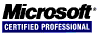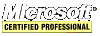# JavaScript Tutorial

## Statements

### The if Statement

The if statement is given below:

``````if (num == 5)
alert("num is equal to 5");					 // single-line statement
else if (num == 7) {
alert("num is equal to 7");					 // block statement
}
else {
alert("num is any value other than 5 and 7"); // block statement
}``````

### The while Statement

The while statement is a pretest loop, which means the escape condition is evaluated before the code inside the loop has been executed. Example `while` loop:

``````var num = 0;
while(num++ < 5){
}``````

### The do-while Statement

The do-while statement is a post-test loop, which means that the escape condition is evaluated after the code inside the loop has been executed. The body of the loop is always executed at least once before the expression is evaluated.

``````var num = 0;
do {
} while (num++ < 3);``````

### The for Statement

The for statement is a pretest loop with the added capabilities of variable initialization before entering the loop and defining post-loop code to be executed.

``````for(var i = 0; i < 3; i++) {
}``````

### The for-in Statement

The for-in statement is a strict iterative statement used to enumerate the properties of an object.

``````for (var propertyName in window) {
document.write(propertyName);
}``````

### The break and continue Statements

The break and continue statements provide better control over the execution of code in a loop. The `break` statement exits the loop immediately thereby forcing execution to continue with the next statement after the loop. The `continue` statement stops execution of the loop and returns execution to the test condition. It does not exit the loop unless the loop test condition is false.

``````var num = 0;
for (var i=1; i < 10; i++) {
if (i % 5 == 0) {
break;
}
num++;
}
``````var num = 0;
for (var i=1; i < 10; i++) {
if (i % 5 == 0) {
continue; // this skips the "num++;" statement
}
num++;
}

### The switch Statement

A switch statement can clean up a complex if-else statement.

``````switch (num) {
case 20:
case 30: